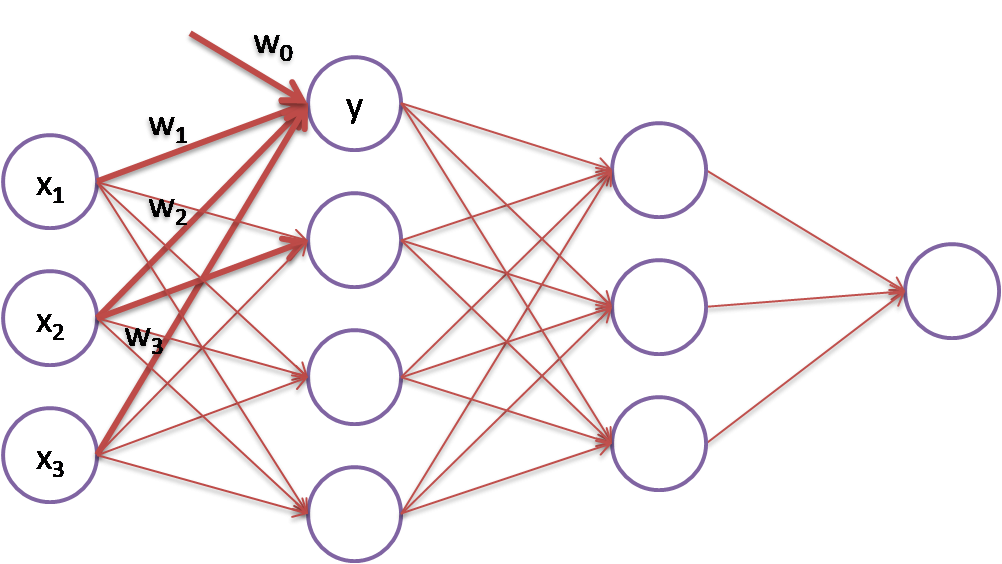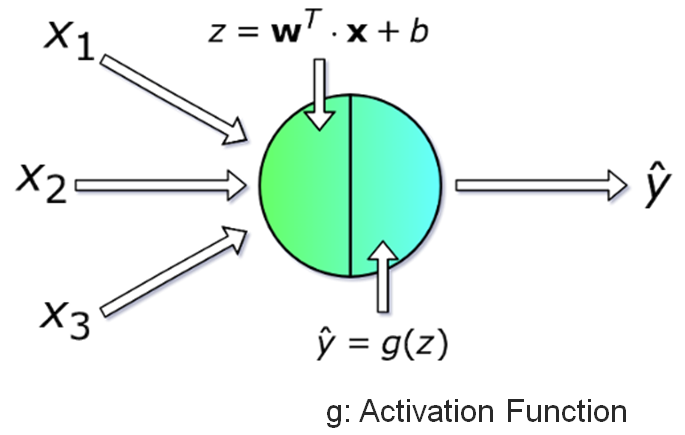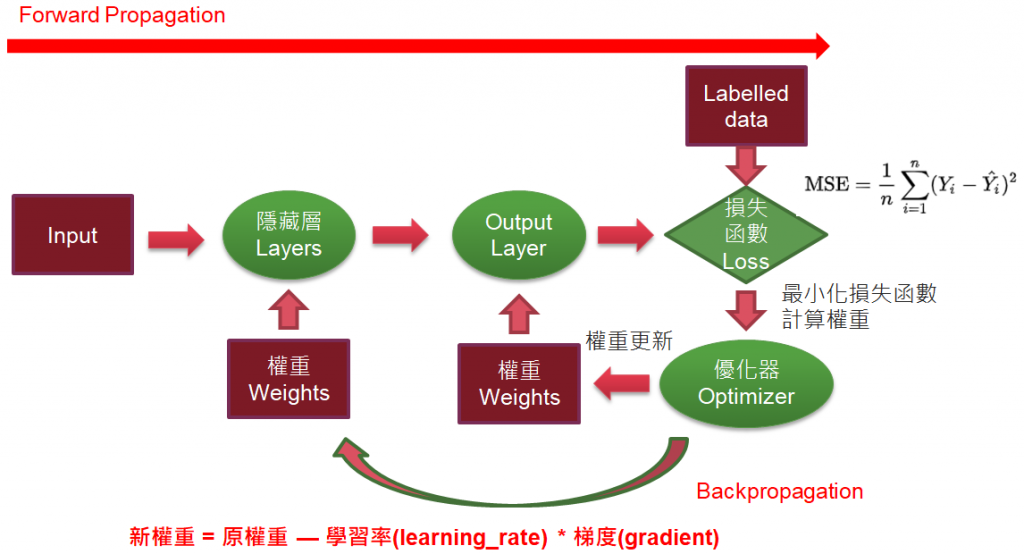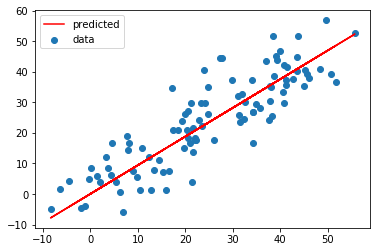#DAY 2
1
AI & Data

## 為什麼需要深度學習的套件?

1. 自動微分(Automatic Differentiation)
2. 各式神經層(Layer)的實踐

【梯度下降】(Gradient Descent)是神經網路(Neural Network)優化求解的主流方法，以下圖為例，我們可以將神經網路看作是多條迴歸線的組合，例如，紅粗線所示就是一條迴歸線，y = w0 + w1 * x1 + w2 * x2 + w3 * x3，【梯度下降】的目標依據X/y的訓練資料，對權重(Wi)求解：1. 一開始Wi設為任意值，已知xi，就可以求得yi。
2. 同理，可以算完同一層的其他迴歸線的yi。
3. 下一層又以上一層的yi為輸入，計算下一層的神經元。
4. 經過層層的計算，最後可以算出預測值，進而求出損失函數，例如均方誤差(MSE)，公式如圖三所示。

2. 再參照下面的公式，更新所有迴歸線的權重:
新權重 = 原權重 — 學習率(learning_rate) * 梯度(gradient)
3. 再回到正向傳導，依據新權重，求算預測值。

## 程式碼

``````import numpy as np
import tensorflow as tf

# x 宣告為 tf.constant，就要加 g.watch(x)
x = tf.Variable(3.0)

# 自動微分
#g.watch(x)
y = x * x

dy_dx = g.gradient(y, x) # Will compute to 6.0

# 轉換為 NumPy array 格式，方便顯示
print(dy_dx.numpy())
``````

## PyTorch 範例

``````import torch

y=x*x

# 反向傳導
y.backward()

``````

## 簡單迴歸範例

``````import numpy as np
import tensorflow as tf

# y_pred = W*X + b
W = tf.Variable(0.0)
b = tf.Variable(0.0)

# 定義損失函數
def loss(y, y_pred):
return tf.reduce_mean(tf.square(y - y_pred))

# 定義預測值
def predict(X):
return W * X + b

# 定義訓練函數
def train(X, y, epochs=40, lr=0.0001):
current_loss=0
# 執行訓練
for epoch in range(epochs):
t.watch(tf.constant(X))
current_loss = loss(y, predict(X))

# 取得 W, b 個別的梯度
dW, db = t.gradient(current_loss, [W, b])

# 更新權重
# 新權重 = 原權重 — 學習率(learning_rate) * 梯度(gradient)
W.assign_sub(lr * dW) # W -= lr * dW
b.assign_sub(lr * db)

# 顯示每一訓練週期的損失函數
print(f'Epoch {epoch}: Loss: {current_loss.numpy()}')

# 產生隨機資料
# random linear data: 100 between 0-50
n = 100
X = np.linspace(0, 50, n)
y = np.linspace(0, 50, n)

# Adding noise to the random linear data
X += np.random.uniform(-10, 10, n)
y += np.random.uniform(-10, 10, n)

# reset W,b
W = tf.Variable(0.0)
b = tf.Variable(0.0)

# 執行訓練
train(X, y)

# W、b 的最佳解
print(W.numpy(), b.numpy())

import matplotlib.pyplot as plt

plt.scatter(X, y, label='data')
plt.plot(X, predict(X), 'r-', label='predicted')
plt.legend()
``````